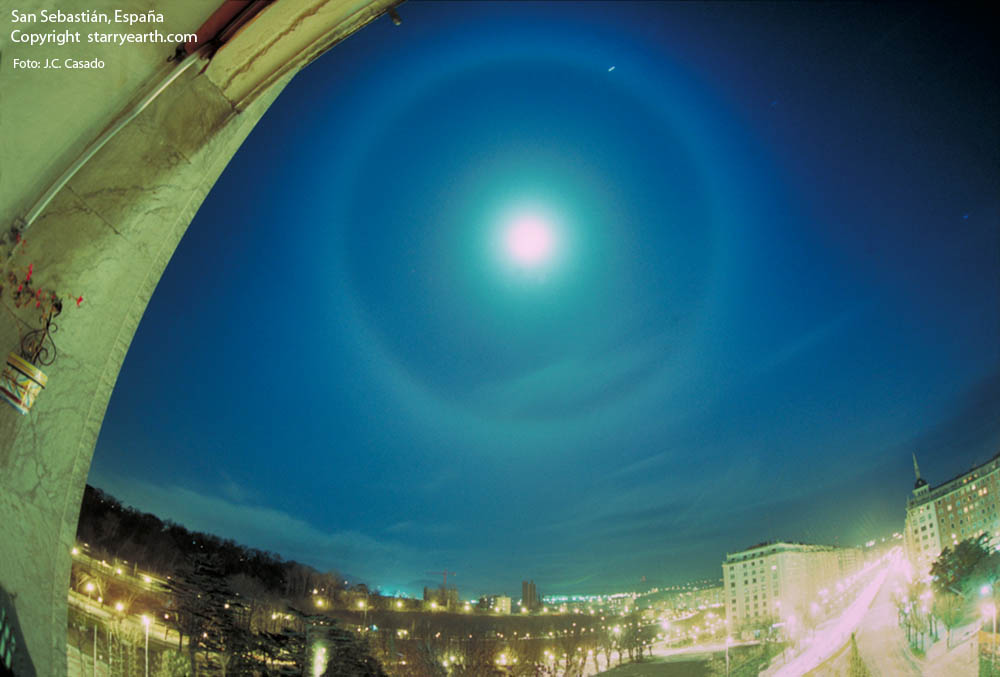## Archive for October, 2011

### tensors

This has been a confusing topic, with half a dozen Wikipedia pages on the subject. Here I took some notes.

Tensors are sums of “products” of vectors. There are different kinds of vector products. The one used to build tensors is, naturally, the tensor product. In the Cartesian product of vector spaces $$V\times W$$, the set elements are tuples like $$(v,w)$$ where $$v\in V, w\in W$$. A tensor product $$v\otimes w$$ is obtained by tupling the component bases rather than the component elements. If $$V$$ has basis $$\{e_i\}_{i\in\{1,…,M\}}$$ and $$W$$ has basis $$\{f_j\}_{j\in\{1,…,N\}}$$, then take $$\{(e_i,f_j)\}_{i\in\{1,…,M\},j\in\{1,…,N\}}$$ as the basis of the tensor product space $$V\otimes W$$. Then define the tensor product $$v\otimes w$$ as

(1) $$\sum_{i,j} v_i w_j (e_i,f_j) \in V\otimes W$$,

if $$v=\sum_i v_i e_i$$ and $$w=\sum_j w_j f_j$$. The entire tensor product space $$V\otimes W$$ is defined as sums of these tensor products

(2) $$\{\sum_k v_k\otimes w_k | v_k\in V, w_k\in W\}$$.

So tensors in a given basis can be represented as multidimensional arrays.

$$V\otimes W$$ is also a vector space, with $$MN$$ basis dimensions (c.f. $$V\times W$$ with $$M+N$$ basis dimensions). But additionally, it has internal multilinear structure due to the fact that it is made of component vector spaces, namely:

$$(v_1+v_2)\otimes w = v_1\otimes w + v_2\otimes w$$
$$v\otimes (w_1+w_2) = v\otimes w_1 + v\otimes w_2$$
$$\alpha (v\otimes w) = (\alpha v)\otimes w = v\otimes (\alpha w)$$Today I looked up at the night sky and there was this wonderfully full moon, but it was sitting in the middle of a huge perfectly round disk opening into the heavens amidst the clouds. I wondered what the heck it was, thinking it might be the result of Earth-shadow.